18 October 2018

# PicoCTF 2018 - Circuit123 WriteUp

by XxcoralloxX

## Circuit123

In this python reverse challenge, we have a flag encoded in sha512 and we need to find the key thanks to a program which check the key before decrypting.

This check is the vulnerability. The program does that to verify “integrity” of the key, and we need to reverse it.

The verify function transform the key into a binary number, reverse it, and used as input for a “simulated circuit”. If the output of the circuit has the right value, the key is considered good.

This “right value”, the circuit and the encrypted flag are in a file called map2.txt Another file called map1.txt is provided to us with the right key to explore the decrypter.

Here’s the code of the verify function. x it’s the key, chalbox it’s the content of the file.

Chalbox is divided into: Lenght (of the key in bit) Gates, a list of logical gates (only or and xor) check, the value that the gate should give as output

In line 8 the key is transformed into bit-sequence and reversed. then for every gate, a new “bit” it’s added to b[] which is a list containing every bit of the circuit.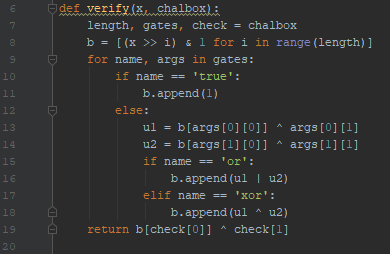in line 23 we can see that it checks if the last bit of the circuit has a specific value (check is an index, check is a boolean, both taken from the file).

## Ok, what’s the point?

The key it’s something like a 128-bit input for this simulated circuit, which has to provide a well know output (0 in the case of map2.txt). We need to reverse the circuit to get what’s the right key.

The file was too hard to read, so I used that verify function to make things easier. Basically, I modify it a bit to make it print all the circuit: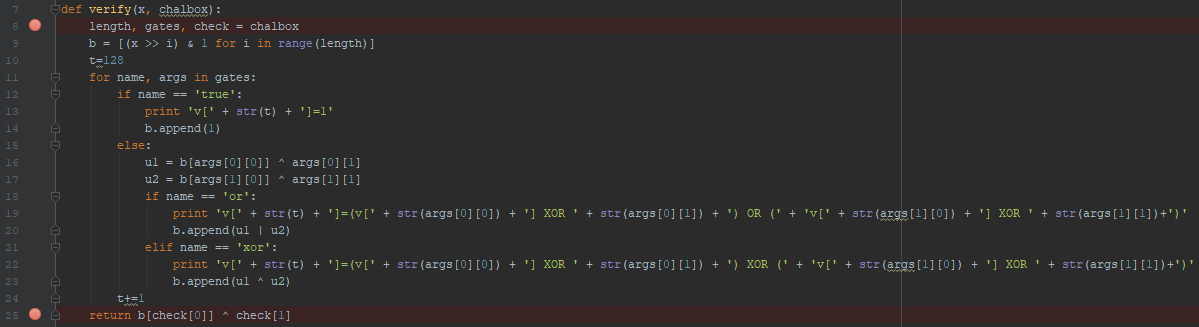A pice of output:

v=1
v=(v XOR False) XOR (v XOR False)
v=(v XOR False) XOR (v XOR True)
v=(v XOR True) OR (v XOR False)
v=(v XOR True) OR (v XOR False)
...
v=(v XOR False) OR (v XOR False)
v=(v XOR False) OR (v XOR False)
v=(v XOR False) OR (v XOR False)


Remember that v[0:127] are the input of the circuit. Debbugging we can also see that v^1 has to be == 1 (Line 25, since check =1140 and check=true for the map2.txt file).

Form this information we can deduce that v=0. And now we should backtrack all the circuit.

## Z3 gets handy

I used a poorly written z3 script in order to do this. First, I rewrote one last time the script, to make it print gates in z3 syntax.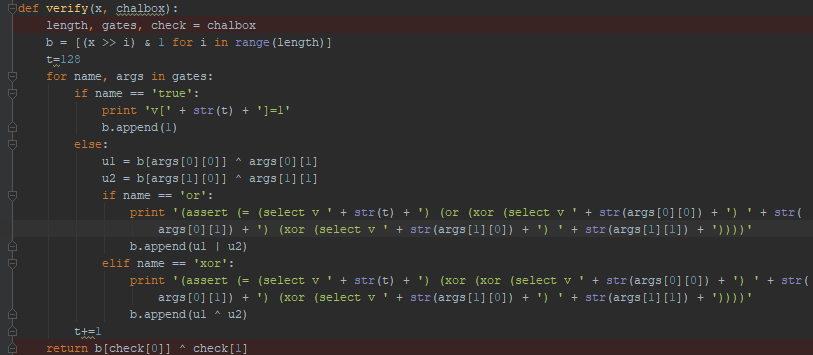Thanks to this output I made this simple z3 script:

https://github.com/pwnthemole/ctfs/blob/master/PicoCTF2018-Circuit123/circuit.smt2

which produce a badly formatted result, here is a pice: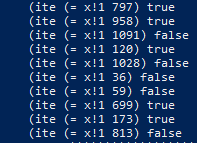(it basically says for every index the boolean value).

Scripting a bit to traduce that in bit (and reversing) we get

“10100101000110110111010001001010000101101110111001000110010101111001011011101111101000101010111101001110000110110011001011001101” which is the key in binary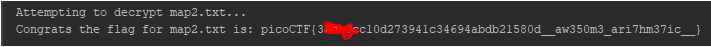This was a nice first approach to z3 to me.

XxcoralloxX

tags: reverse  z3  python  PicoCTF2018